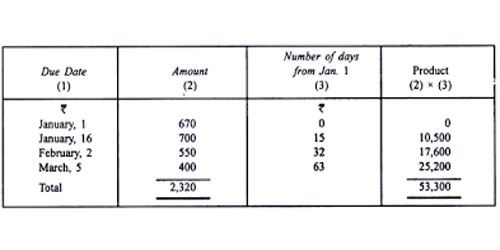Finance

# Average Due DateAverage Due Date

An Average Due Date is an equated date on which a single payment (in place of several payments) may be made of amounts due on different dates. It is the date on which several debts due on different dates can be paid by a single payment without any loss of interest either to debtor or creditor. This method of payment is used for settling payments of Bills of Exchange, which are due for payment on different dates. For example – a person pays all his dues on a particular date, in a manner so that neither the debtor nor the creditor suffers loss or gain by way of interest. This date is the Average due date (ADD).

In a business, it is quite normal to borrow several sums of money from the same lender. Sometimes, to ease the payments, we calculate an average due date so that all payments can be made on one date. There are two alternatives available in such a case: (a) Interest may be calculated separately for each transaction; or (b) A mean date may be determined and the interest may be calculated from such mean date to the date of actual settlement on the total amount due by one party to another.

Average due date = Base date ± [Total of the products/Total of the amounts]

It is calculated in the following manner:

• Fix one of the due dates as the base date.
• Calculate the number of days of each one of the other due dates away from the base date.
• Multiply each amount by its respective number of days as calculated in the second step.
• Add the amounts and the products separately.
• Divide the sum of products by the total of the amounts. This gives the number of days the average due date is away from the base date.
• Add the number of days to the base date and thus arrive at the average due date.

The utility of Average Due Date:

• problems relating to the calculation of interest on drawings by partners, on different dates.
• the settlement of accounts by a series of bills of exchange due on different dates.
• the settlement of accounts between one trader and another or a trader and his customers.